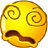Keith wrote:

> Can't we reduce \$$\mathcal{V}\$$-functors to changes-of-base, mainly a change-of-base between a \$$\mathcal{V}\$$-category and itself?

No, you're making a classic level slip here. There's _no such thing_ as change-of-base between a \$$\mathcal{V}\$$-category and itself! Change-of-base is not a kind of map between \$$\mathcal{V}\$$-categories.

It's like "a function from an element of a set to itself."

There's _no such thing_ as a function from an element of a set to itself. A function \$$f: X \to Y\$$ goes between _sets_ \$$X\$$ and \$$Y\$$. If \$$X = Y\$$ this function may _map_ an element \$$x \in X\$$ to itself: \$$f(x) = x\$$. But it's still a function from \$$X\$$ to \$$X\$$, not a function from \$$x\$$ to \$$x\$$.

Similarly, there's _no such thing_ as a change-of-base from a \$$\mathcal{V}\$$-category to itself. Change-of-base turns \$$\mathcal{V}\$$-categories into \$$\mathcal{W}\$$-categories, so it's a functor \$$\Phi: \mathcal{V}\mathrm{Cat}\to \mathcal{W}\mathrm{Cat} \$$. If \$$\mathcal{V} = \mathcal{W}\$$ this functor may _map_ a \$$\mathcal{V}\$$-category \$$\mathcal{C}\$$ to itself: \$$\Phi(\mathcal{C}) = \mathcal{C}\$$. But it's still a functor from \$$\mathcal{V}\mathrm{Cat}\$$ to \$$\mathcal{V}\mathrm{Cat}\$$, not an enriched functor from \$$\mathcal{C}\$$ to \$$\mathcal{C}\$$.

This is why change-of-base and enriched functors are both crucial, indispensable concepts.

I've been through this confusion myself a couple of times! It only takes 8 hours of agony to figure out what's going on.Let me review the whole story:

First I told you about enriched functors between \$$\mathcal{V}\$$-categories. They're called \$$\mathcal{V}\$$-functors.

Then I told you about enriched functors from a \$$\mathcal{V}\$$-category to a \$$\mathcal{W}\$$-category. But these only makes sense when you first have a trick for turning \$$\mathcal{V}\$$-categories into \$$\mathcal{W}\$$-categories. This trick is "change of base".

Suppose \$$\mathcal{V}\$$ and \$$\mathcal{W}\$$ are monoidal posets and \$$f: \mathcal{V} \to \mathcal{W}\$$ is a monoidal monotone. Suppose \$$\mathcal{X}\$$ is a \$$\mathcal{V}\$$-category. Then there's a \$$\mathcal{W}\$$-category \$$\Phi(\mathcal{X})\$$ with the same objects as \$$\mathcal{X}\$$ and

$\Phi(\mathcal{X})(x,x') = f(\mathcal{X}(x,x')).$

This trick, \$$\Phi\$$, is called **change of base**.

Once you've got change of base, you can get an enriched functor from a \$$\mathcal{V}\$$-category \$$\mathcal{X}\$$ to a \$$\mathcal{W}\$$-category \$$\mathcal{Y}\$$. First you use change of base to turn \$$\mathcal{X}\$$ into a \$$\mathcal{W}\$$-category \$$\Phi(\mathcal{X})\$$. Then you pick a \$$\mathcal{W}\$$-functor

$F : \Phi(\mathcal{X}) \to \mathcal{Y} .$

In short: _first you move \$$\mathcal{X}\$$ to the world where \$$\mathcal{Y}\$$ lives, and then it can talk to \$$\mathcal{Y}\$$_.